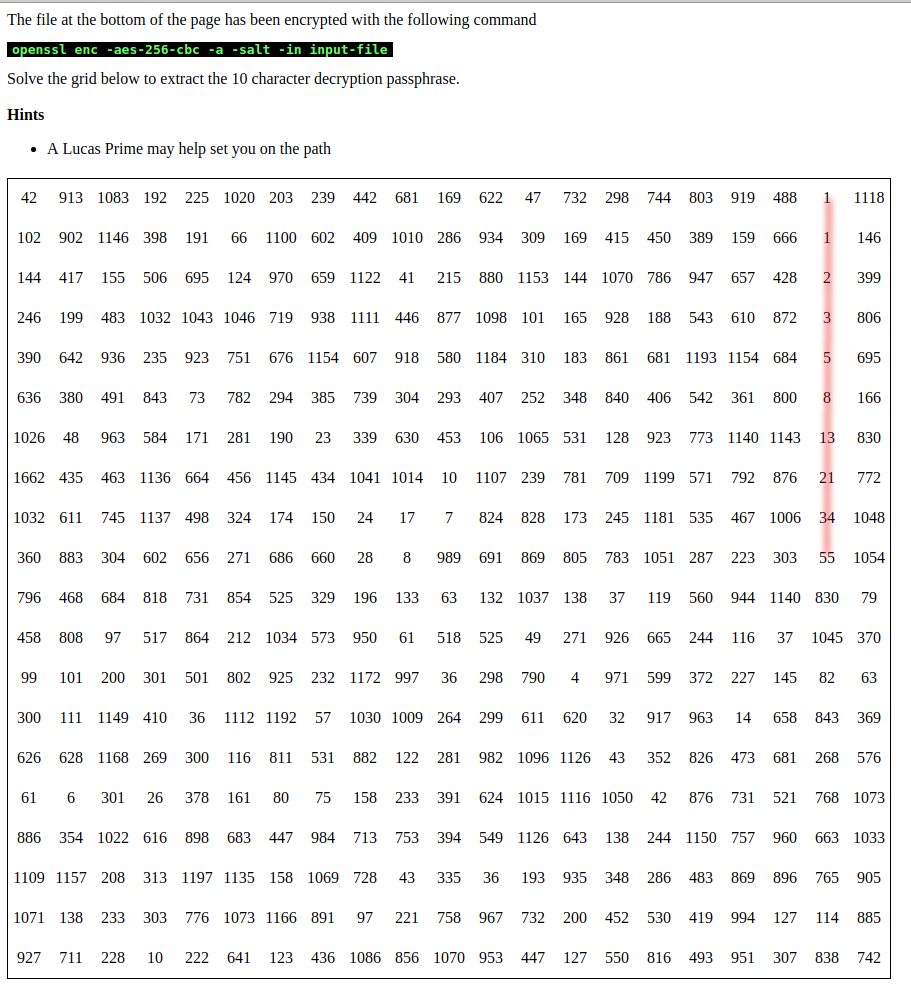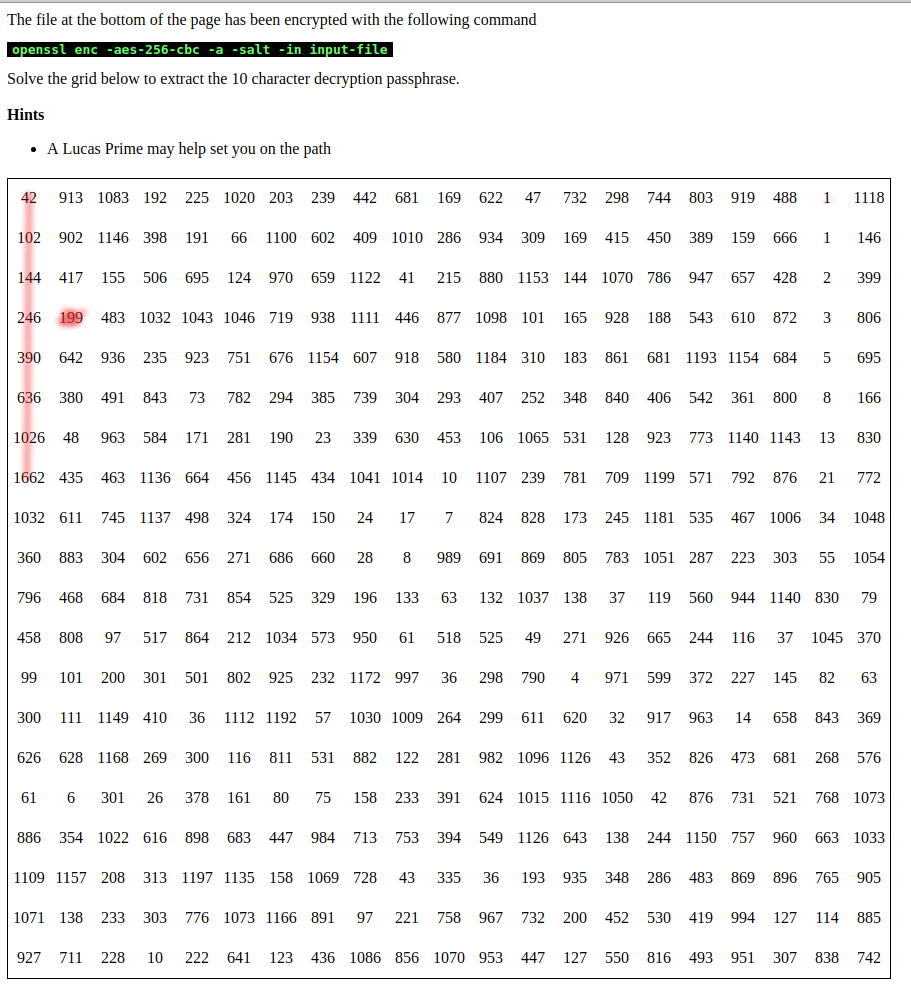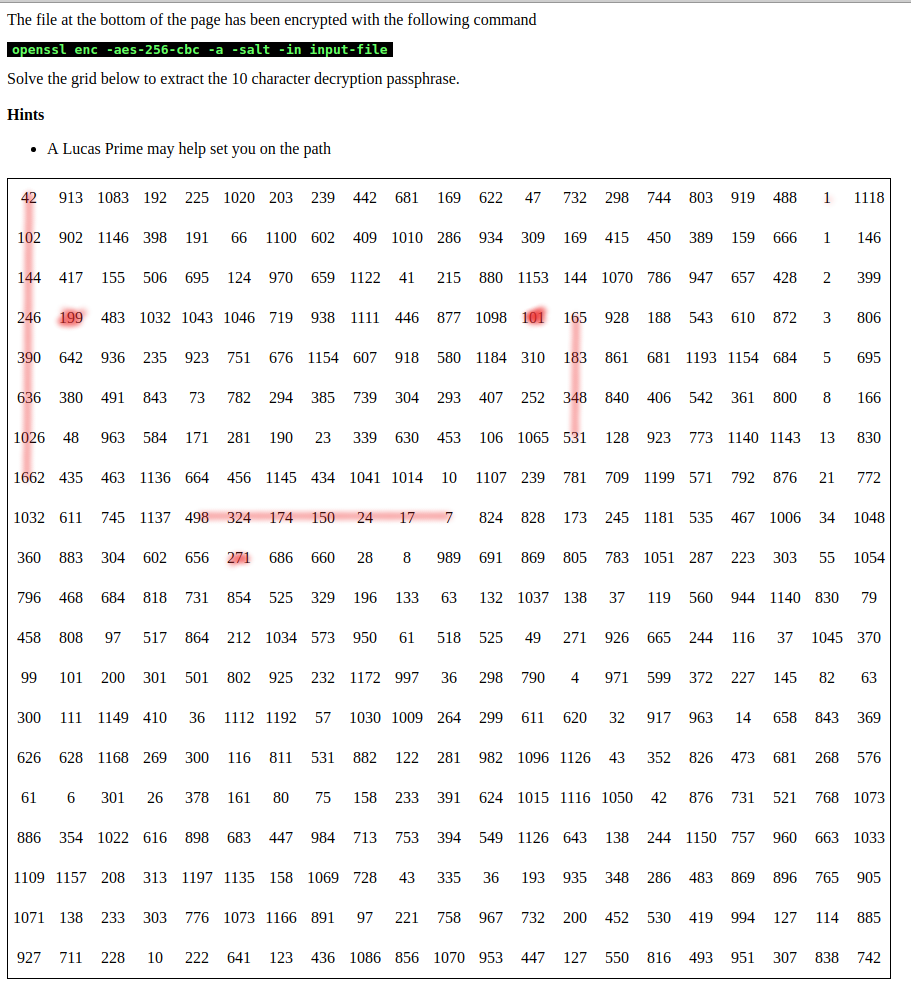# Solution to my April 2016 Puzzle

It's been three years now, and although I've had many people complain about it giving them a headache, to my knowledge no-one has solved the puzzle I posted in April 2016. My other puzzles and crypto trails have all fallen in significantly less time, but I've watched people really struggle with this one, so I think it's fair to say that I made it just a little too hard.

It only seems fair, therefore, to explain the solution (while I can still remember it).

This post will do just that (there's a video of solving it below for those who don't want to read)

### The Puzzle

The puzzle presents you with the command used to encrypt a file, a number grid, and the ascii armoured encrypted file.The challenge is to solve the grid in order to calculate the encryption key.

### Solving the puzzle

The first step, as with any number grid is to look for patterns. The eagle eyed will quite quickly spot a fibonacci sequence running down the right hand sideThis sequence itself isn't actually used, but hopefully this and the reference to a Lucas prime (Lucas also being a fibonnacci like sequence) should alert you to the possibility there may be other fibonacci like sequences. In fact, you'll find one right next to a Lucas primeSo we've got a fibonacci style sequence adjacent to a prime. This also occurs elsewhere in the grid, and the sequences don't always run forwardsIt's more than possible to sit and identify the sequences manually, but it's far easier to write some code to find them for you. Ultimately you'll end up with some code which solves the entire puzzle (see the bottom of this post for python and javascript solutions).

The next thing to do is to work out what to do with the identified sequences. The adjacent prime obviously has some relevance as the actual fibonacci sequence doesn't have one.

A bit of trial and error should reveal that if you subtract the prime from the number it's next to (e.g. 246-199) you'll always get a number in the range 0-127. This, conveniently is also the ASCII number range, so 246-199=47 which is the character /.

So, reading left to right, we can now assemble a 10 character string, at which point we've got our decryption passphrase.

It's then simply a case of dumping the ciphertext into a file, and then running

``````openssl enc -aes-256-cbc -a -d -salt -in [file]
``````

Providing the decryption passphrase when prompted.

### Solution Code

Javascript

As shown in the video above, it's sufficient to open developer tools and paste into the console. It'll also colour the relevant cells to show how it reached the solution

``````var css=document.createElement('style');
css.type='text/css';
css.innerHTML='.detectedPrime {background-color: red;} .sequenceMember {background-color: blue} .selected {background-color: yellow}';
var ele,eles,pele,ref,prime,sno, nums = {},ks =[],primes=[],neighbours=[],selecs=[],neighbours=[],identifiedPairs=[];

// Check whether a number is a prime
function checkNumPrime(n){

// Check whether the number is 1,2 or 3
if (n<4){
return true;
}

// Check the number isn't directly divisible by 2 or 3
if (n%2 == 0 || n%3 == 0){
return false;
}

var di=2;
var i = 5;

// Don't calculcate higher than the square root (rounded down if needed)
var lim = Math.floor(Math.sqrt(n));

while (i < lim){

if (n%i == 0){
return false;
}
i=i+di;
di=6-di;
}

// If we haven't already returned, n is prime
return true;

}

function decodeReference(id){
// Remove the r
id=id.substr(1);
return id.split('c');

}

// Calculate the DOM ID of all adjacent cells
function calculateNeighbours(id){

if (!id){
return [];
}

// Break the cell ID into a reference array
var reference = decodeReference(id);

// Calculate all adjacent neighbours by subtracting and adding to the reference
var neighbours=[];

// Calculate the permutations
var incr = parseInt(reference)+1;
var incc = parseInt(reference)+1;

var decr = parseInt(reference)-1;
var decc = parseInt(reference)-1;

// Don't use negative numbers
if (decr >= 0){
neighbours.push("r"+decr+"c"+reference); // r-1,c
}else{
neighbours.push(false);
}

// And don't fall off the edge of the grid
if (incr <20){
neighbours.push("r"+incr+"c"+reference); // r+1,c
}else{
neighbours.push(false);
}

if (decc >=0){
neighbours.push("r"+reference+"c"+decc); // r,c-1
}else{
neighbours.push(false);
}

if (incc <20){
neighbours.push("r"+reference+"c"+incc); // r,c+1
}else{
neighbours.push(false);
}

return neighbours
}

// dir should be either c (column) or r (row)
function getDirectionalNeighbours(id,dir){

var neighbours = calculateNeighbours(id);
var dirneighbours = []

if (dir == 'c'){
dirneighbours.push(neighbours);
dirneighbours.push(neighbours);

}else{
dirneighbours.push(neighbours);
dirneighbours.push(neighbours);
}

return dirneighbours;
}

// Check Cells and identify whether they're part of a sequence
function isPartOfSequence(c1,c2,c3){

if (!c1 || !c3){
// don't fall off the end of the grid

// Array is - isSequence, checked, sequenceorder, [cell references]
return [false,false,false, [c1,c2,c3]];
}

n1 = parseInt(document.getElementById(c1).innerHTML);
n2 = parseInt(document.getElementById(c2).innerHTML);
n3 = parseInt(document.getElementById(c3).innerHTML);

var seq = false;
var order = false;
var cells = [c1,c2,c3];
// Sequences can be ascending or descending, so we need to test both
if (n1 + n2 == n3){
seq = true;
order = 'asc';
}

if (n1 - n2 == n3){
seq = true;
order = 'desc';
}

return [seq,true,order,cells];
}

function colourSequenceMembers(ids){

for (var i=0; i<ids.length;i++){
document.getElementById(ids[i]).className = 'sequenceMember';
}

}

function enforceFourWay(cells,direction){
var dnb = getDirectionalNeighbours(cells,direction);
var dseq = isPartOfSequence(dnb,cells,dnb);
var match = false;

if (dseq == true){
colourSequenceMembers(dseq);
match=true;
}

var unb = getDirectionalNeighbours(cells,direction);
var useq = isPartOfSequence(unb,cells,unb);
if (useq == true){
colourSequenceMembers(useq);
match=true;
}
return match;
}

// Becaues Javascript is a dick when it comes to sorting numbers
function sortNumber(a,b) {
return a - b;
}

// Cycle through cells and colour the primes as a starting point
eles = document.getElementsByTagName('td');

for (var i=0; i<eles.length; i++){
if (checkNumPrime(eles[i].innerHTML)){
primes.push(eles[i].id);
}
}

// Work over each prime and identify it's neighbours, then look for sequences
for (var i=0; i<primes.length; i++){
neighbours=calculateNeighbours(primes[i]);

// Check for sequences in neighbours
for (var r=0; r<neighbours.length; r++){
if (! neighbours[r]){
// Don't check anything that returned false
continue;
}

var direction = 'r';

if (r>1){
direction = 'c';
}

// Get the cell's directional neighbours
cnumbers=getDirectionalNeighbours(neighbours[r],direction);

// Test if it's part of a sequence (passing cell references in the order they appear in the table
seq = isPartOfSequence(cnumbers,neighbours[r],cnumbers);

if (seq == true){
// We found a Fibonnaci like sequence!
// But, the rules (now) say each sequence must be > 3 cells, so we need to check for that
if (enforceFourWay(seq,direction)){
colourSequenceMembers(seq);
selecs.push([neighbours[r],primes[i]]);
}

}else{
// Check the neighbours neighbours - just in case the prime is sat at the end of a sequence
nnumbers=getDirectionalNeighbours(cnumbers,direction);
seq = isPartOfSequence(nnumbers,cnumbers,nnumbers);

if (seq == true){
// We found a Fibonnaci like sequence!

// Apply the 4 cell rule
if (enforceFourWay(seq,direction)){
colourSequenceMembers(seq);
selecs.push([neighbours[r],primes[i]]);
}
}

// And the other direction
nnumbers=getDirectionalNeighbours(cnumbers,direction);
seq = isPartOfSequence(nnumbers,cnumbers,nnumbers);

if (seq == true){
// We found a Fibonnaci like sequence!

// Apply the 4 cell rule
if (enforceFourWay(seq,direction)){
colourSequenceMembers(seq);
selecs.push([neighbours[r],primes[i]]);
}

}
}

}

}

// Work through and start calculating char codes
for (i=0; i<selecs.length; i++){
ele = document.getElementById(selecs[i]);
pele = document.getElementById(selecs[i]);
ele.className='selected';
pele.className = 'detectedPrime';

prime=parseInt(pele.innerHTML);
sno=parseInt(ele.innerHTML);
computedchar = sno - prime;
// Discard anything not resulting in a printable asci char
if (computedchar > 127 || computedchar < 32){
continue;
}

// Numbers should be read left to right, so we need to get column number ready for sorting later
ref = decodeReference(selecs[i]);

ks.push(ref);
nums["c"+ref] = computedchar;
}

// Sort the columns into Left -> Right order
ks.sort(sortNumber);

// Work through and print the characters in order
s='';

for (var i=0; i<ks.length; i++){
s += String.fromCharCode(nums['c'+ks[i]]);
}

``````

Python

``````
#!/usr/bin/env python
#
# Automatically solve puzzle-201604 and recover the password
#
# Pass the script any argument on the commandline to have it automatically decrypt the bounty
#
#

import requests, math,sys
from lxml import etree

primes = []
selecs = []
ks = {}
passphrase = []
pagecontent = False
column = 0

def fetchPage():
''' Get the page contents
'''
global pagecontent
if not pagecontent:
Page = etree.HTML(r.content)
return Page

def fetchPuzzle():
''' Extract the puzzle table from the DOM
'''
tree = fetchPage()
return tree.xpath('//table//tr')

def fetchFile():
''' Get the bounty file from the DOM
'''
tree = fetchPage()
return tree.xpath('//pre[@id="filecontents"]//text()')

def checkNumPrime(n):
''' Check whether n is a prime number
'''

# Numbers less than 4 are all prime
if n < 4:
return True

# Numbers divisible by 2 or 3 aren't prime
if n%2 == 0 or n%3 == 0:
return False

# Create the seeds
di=2
i=5

# Work out the square root of n (no need to check anything higher)
lim = math.floor(math.sqrt(n))

while i < lim:
if n%i == 0:
return False

i=i+di
di=6-di

# If we got this far, didn't find a whole number. Number is prime
return True

def getCellValueById(cellid):
''' The equivalent of doing document.getElementById(cellid).innerHTML in JS
'''
global puzzletable
return puzzletable.xpath('//tr//td[@id="'+cellid+'"]//text()');

def decodeReference(cell):
''' Break a cell ID into a dict containing row and column
'''
cell = cell[1:]
return cell.split('c')

def calculateNeighbours(cell):
''' Calculate the IDs of neighbouring cells
'''
if not cell:
return False

reference = decodeReference(cell)
neighbours = []

incr = int(reference) +1
incc = int(reference) +1
decr = int(reference) - 1
decc = int(reference) - 1

# Prevent negatives - we don't want to fall off the grid
if decr >= 0:
neighbours.append("r"+str(decr)+"c"+reference)
else:
neighbours.append(False)

if incr < 20:
neighbours.append("r"+str(incr)+"c"+reference)
else:
neighbours.append(False)

if decc >= 0:
neighbours.append("r"+reference+"c"+str(decc))
else:
neighbours.append(False)

if incc < 20:
neighbours.append("r"+reference+"c"+str(incc))
else:
neighbours.append(False)

return neighbours

def getDirectionalNeighbours(cellid,direction):
''' Get neighbours moving either longitudinally (c) or latidudally (r)
'''

# Shouldn't actually happen, but be safe
if not cellid:
return [False,False,False,False]

neighbours = calculateNeighbours(cellid)
dirneighbours = []
if direction == "c":
dirneighbours.append(neighbours)
dirneighbours.append(neighbours)
else:
dirneighbours.append(neighbours)
dirneighbours.append(neighbours)

return dirneighbours

def isPartOfSequence(c1,c2,c3):
''' Check whether cells form part of a sequence - Fn = Fn-1 + Fn-2
'''
if not c1 or not c3:
return [False,False,False,[c1,c2,c3]]

n1 = int(getCellValueById(c1))
n2 = int(getCellValueById(c2))
n3 = int(getCellValueById(c3))

seq = False
order = False
cells = [c1,c2,c3]

# Sequences can be asc or desc, test both

if n1 + n2 == n3:
seq = True
order = 'asc'
elif n1 - n2 == n3:
seq = True
order = 'desc'

return [seq,True,order,cells]

def enforceFourWay(cells,direction):
''' Enforce the four cell rule
'''
dnb = getDirectionalNeighbours(cells,direction)
dseq = isPartOfSequence(dnb,cells,dnb)
if not dseq:
# Check the other end of the sequence we found
unb = getDirectionalNeighbours(cells,direction)
return isPartOfSequence(unb,cells,unb)

return True

def asint(s):
''' Stackoverflow poach - helps sort a dictionary numerically
'''
try: return int(s), ''
except ValueError: return sys.maxint, s

def DecryptFile(pw):
''' Take the recovered password and decrypt the bounty
'''
import tempfile,os
from pipes import quote
from subprocess import check_output

filecontent=fetchFile()
tmphandle,tmpfile = tempfile.mkstemp()
f = open(tmpfile,'w')
f.write(filecontent)
f.close()
os.close(tmphandle)
cmd = "openssl enc -aes-256-cbc -a -d -salt -k "+quote(pw)+" -in "+tmpfile
output = check_output(cmd,shell=True)
os.remove(tmpfile)
return output

if __name__ == '__main__':
try:
if sys.argv:
decrypt=True
except:
decrypt=False

puzzletable = fetchPuzzle()
# Pick the primes out of the table by iterating over the cells
while column < 20:
row = 0
while row < 20:
cellref='r'+str(row)+'c'+str(column)
if checkNumPrime(int(getCellValueById(cellref))):
primes.append(cellref)
row = row +1
column = column + 1

# Work over each prime and identify it's neighbour
for prime in primes:
neighbours = calculateNeighbours(prime)
# Check neighbours for sequences
for r,neighbour in enumerate(neighbours):
if not neighbour:
continue

direction = "r"

if r > 1:
direction = "c"

# Get the cells directional neighbours
cnumbers = getDirectionalNeighbours(neighbour,direction)

# Check for a sequence in those neighbours
seq = isPartOfSequence(cnumbers,neighbour,cnumbers)
if seq:
# We found a matching sequence, need to make sure there's >= 4 cells in the sequence
if enforceFourWay(seq,direction):
selecs.append([neighbour,prime])
else:
# Check the neighbour's neighbours - the prime might be sat on the last in a sequence
nnumbers=getDirectionalNeighbours(cnumbers,direction)
seq = isPartOfSequence(nnumbers,cnumbers,nnumbers)
if seq:
# Fourway
if enforceFourWay(seq,direction):
selecs.append([neighbour,prime])

# Now check in the other direction
nnumbers=getDirectionalNeighbours(cnumbers,direction)
seq = isPartOfSequence(nnumbers,cnumbers,nnumbers)
if seq:
# Fourway
if enforceFourWay(seq,direction):
selecs.append([neighbour,prime])

# Start calculating the char codes
for select in selecs:
ele = int(getCellValueById(select))
pele = int(getCellValueById(select))

computed = ele - pele
# Skip non printable ASCII Char codes
if computed > 127 or computed < 32:
continue

# Numbers need to be decoded left to right, so we still care about cell ids
ref = decodeReference(select)
ks[str(ref)] = computed

# Sort into column order
for key in sorted(ks,key=asint):
passphrase.append(chr(ks[key]))

pw = ''.join(passphrase)